## Abstract

Wireless Sensor Network (WSN) is widely used in the industrial Network Control System (NCS). It relies on the IEEE 802.15.4 MAC protocol to transfer data at low rate. Experimental and theoretical studies proved that the wireless channel produces a time delay. In the NCS, this delay may cause system instability and performance degradation. Delay compensation schemes are used to reduce the delay effects.

In this paper, a delay compensation scheme using classical and adaptive Smith predictor is applied to wireless NCS. The Markov model is proposed to compute the estimated network delay used in the classical predictor. In the adaptive predictor, the channel delay statistics using shift register is proposed to update the estimated delay. To evaluate the proposed schemes, a DC-motor controller system based on IEEE 802.15.4 is built using True Time Matlab software. The system performance with and without the proposed delay compensation scheme is studied. It is also compared to other delay compensation schemes. The results show that the proposed scheme improves the NCS performance significantly and reduces the effect of the delay on the system.

## Keywords

Networked control systems; IEEE 802.15.4 MAC; Time delay compensation; Smith predictor

## 1. Introduction

Networked Control Systems (NCSs) are widely used in the industrial applications . The traditional NCS consists of sensors, and actuators directly wired to the controllers. In recent NCSs, the wired network is replaced by wireless network that is easier in installation and maintenance. The wireless network transfers the data from the sensor to the controller and from the controller to the actuator at low data rate. This network requires a protocol designed for low data rate, low power consumption and low computational complexity.

IEEE 802.15.4 protocol meets the requirements of the wireless NCS ,  and . IEEE 802.15.4 medium access control (MAC) protocol supports the non-beacon and beacon modes and it uses the carrier sensing multiple access/collision avoidance (CSMA/CA) mechanism for conflict resolution and positive acknowledgments for successfully received packets. To quantify the network performance of this protocol, Markov chain model is applied to model the IEEE 802.15.4 MAC protocol ,  and . The model is also used to derive some performance metric such as throughput, delay, power consumption, and packet discard probability . This study proved that the IEEE 802.15.4 MAC protocol produces a transmission delay measured as the number of slots it takes from the moment the packet reaches the head of the line at the sender to the moment it arrives at its destination.

In the NCS, any time delay results in performance degradation and can cause instability. This delay is a function of devices’ processing times and transmission delay due to network protocols used in the systems. To reduce the effect of time delay, delay compensation schemes for wired NCS are proposed in ,  and . In  the scheme is based on observer derived in it. In , the scheme is based on design controller using the well-known direct synthesis method and implemented in a didactic networked control system platform. In , the scheme is based on classical and adaptive Smith predictors, and it is applied to wired NCS (Ethernet). The used induced time delay was random value in the case of classical Smith predictor, and the measured network delay in the case of adaptive Smith predictor. Delay compensation schemes for wireless NCS are proposed in  and . In , the scheme is based on adaptive Smith predictors. In , communication disturbance observer (CDOB) and network disturbance (ND) have been introduced to compensate variable delay in communication link for NCS. There are also other schemes of delay compensation, such as optimal controller, Fuzzy controller, robust control and sliding mode controller.

This paper proposes a delay compensation scheme using classical and adaptive Smith predictor to the wireless NCS (IEEE 802.15.4). In the classical Smith predictor, the induced time delay is computed based on the Markov model of the IEEE 802.15.4 network. The adaptive Smith predictor is modified to consider the network statistics, so the delay is computed as the average of the measured network delays. To evaluate the performance of the proposed scheme, a DC-motor controller system is built using the TrueTime MATLAB software tool . A comparison between the system performance with and without delay compensation scheme is presented. The effect of the number of sensors on the network delay is also studied.

The paper is organized as follows. Section 2 introduces the NCS and their types. Section 3 presents the delay compensation scheme using Smith predictor. Section 4 presents classical Smith predictor and the computation of the induced time delay using Markov model. Section 5 presents the adaptive smith predictor. Section 6 presents the DC-motor speed control system using IEEE 802.15.4. It also shows and discusses the simulation results. Section 7 concludes the paper and presents ideas for future work.

## 2. Networked control systems

Networked Control System (NCS) is a closed loop control system and it consists of sensors, actuators, controllers and a shared network to transfer information among the other control system components.

NCSs can be classified into shared-network control systems and remote control systems. In the shared-network control systems, the shared-network resources are used to transfer measurement signals, from sensors to controllers, and control signals from controllers to actuators. These systems are systematic and structured, and provide more flexibility in installation, and easier maintenance and troubleshooting. On the other hand, in the remote control system, the sensors and actuators are called a plant and their location is called “remote site”. A central controller is installed far away from the sensors and actuators, and its place is typically called a “local site”. This type includes remote data acquisition systems and remote monitoring systems.

To design a NCS, there are two general approaches shown in Fig. 1. The first approach is the hierarchical structure consisting of central controller and subsystems containing a sensor, an actuator, and a controller. In this case, when the subsystem controller receives a set point from the central controller, the subsystem tries to satisfy this set point by itself. The measurement signal is transmitted via a network to the central controller. The second approach is the direct structure where the plant (sensors and actuators), and a controller are connected directly to a network. In this approach, each plant has its corresponding controller.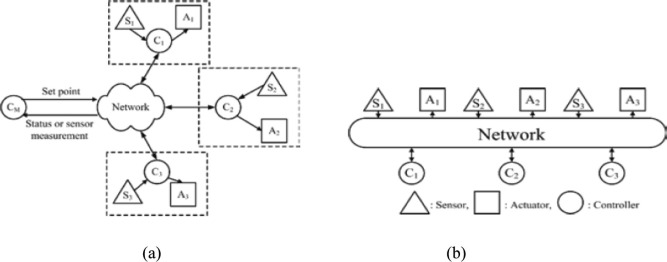Figure 1. Design of network control system: (a) Hierarchical structure, (b) Direct structure.

In this paper, the direct structure design approach is applied to build a DC-motor speed control NCS. The shared network is the IEEE802.15.4 wireless network.

## 3. Delay compensation using smith predictor

Wireless NCS is considered as a closed-loop system consisting of a plant (the sensors and the actuators) and the controller. These components are communicating using the shared network IEEE 802.15.4. Since the studies showed that the wireless network produces time delay, the network can be modeled as time-delay. A simplified NCS block diagram is shown in Fig. 2, where ${\textstyle t_{1}}$ is the command delay time from the controller to the actuator, ${\textstyle t_{2}}$ is the feedback delay time from the sensor to the controller, ${\textstyle G_{c}(s)}$ is the transfer function of the controller and ${\textstyle G_{p}(s)}$ is the transfer function of the real plant without the delay time. The total time, denoted ${\textstyle t_{p}}$, is the summation of the command delay and the feedback delay ${\textstyle \left(t_{p}=t_{1}+t_{2}\right)}$. The transfer function of the system is given by

 ${\frac {Y(s)}{R(s)}}={\frac {G_{c}(s)G_{p}(s)e^{-t_{1}s}}{1+G_{c}(s)G_{p}(s)e^{-(t_{1}+t_{2})s}}}$
(1)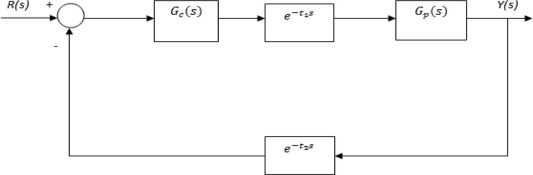Figure 2. The simplified block diagram of NCS.

To reduce the effect of the total delay, the Smith predictor is applied as shown in Fig. 3. The transfer function for the system is given as

 ${\frac {Y(s)}{R(s)}}={\frac {G_{c}(s)G_{p}(s)e^{-t_{1}s}}{1+{\overset {\mbox{ˆ}}{G}}_{p}(s)G_{c}(s)(1-e^{-t_{m}s})+G_{c}(s)G_{p}(s)e^{-(t_{1}+t_{2})s}}}$
(2)

where ${\textstyle t_{m}}$ is the nominal delay time adopted for the Smith predictor and ${\textstyle {\overset {\mbox{ˆ}}{G}}_{p}(s)}$ is the nominal model for the system. With an accurate model of the plant and the time-delay, ${\textstyle {\overset {\mbox{ˆ}}{G}}_{p}(s)=G_{p}(s)}$, and ${\textstyle t_{m}=t_{p}}$. The transfer function can be simplified as follows:

 ${\frac {Y(s)}{R(s)}}={\frac {G_{c}(s)G_{p}(s)}{1+G_{c}(s)G_{p}(s)}}e^{-t_{1}s}$
(3)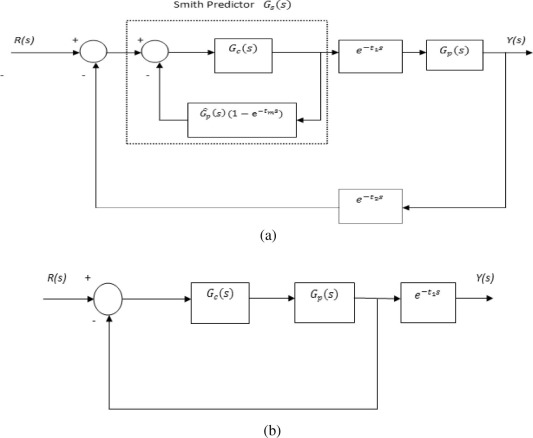Figure 3. The system with the smith predictor: (a) The original system; (b) The equivalent system.

Eq. (3) shows that the complicated transfer function of the delay time becomes two simple parts. One part is the transfer function of the system without the delay time, and the other is the pure simple delay. The equivalent block diagram of Eq. (3) is shown in Fig. 3(b). The system presents the same closed-loop system but only with the pure command (forward) delay time as t1.

## 4. Classical Smith predictor

Classical smith predictor considers tm as a constant value, and its value is assigned randomly in . To estimate accurate tm value, this paper proposes to compute tm based on the IEEE 802.15.4 Markov model.

Markov chain is widely used to model the IEEE 802.15.4 MAC protocol in order to understand the network behavior and quantify its performance ,  and . In the Markov model, a network consists of N nodes working in the saturated case and in Acknowledgment (ACK) mode with retransmission. The saturated case means that each node always has a packet available for transmission. The packet transmitted after a successful channel access procedure can either be received successfully or experience a collision. In the ACK mode with retransmissions, a successful transmission is accompanied by the reception of an ACK, which has a fixed length of Lack backoff periods. The ACK is fed back to the sender node after a minimum time which allows the transceiver of the node to switch from transmission mode (TX) to reception mode (RX). The ACK is expected by the sender node to be received before a fixed maximum time of macAckWaitDuration. If the ACK is not correctly received after this time, a collision is declared. A collided packet can be retransmitted at most R = aMaxFrameRetries times if required, before being discarded. All data packets have the same size L (measured in number of slots) and that their transmission takes an integer multiple of the back off period.

Each node applies the slotted CSMA/CA mechanism, and maintains three variables namely the number of backoff stage (NB), the contention window length (CW) and the backoff exponent (BE). The BE value must satisfy macMinBE ⩽ BE ⩽ macMaxBE. Before every new transmission, the three variables are initialized by 0, 2 and macMinBE, respectively. The node waits for a random number of back off periods specified by the back off value drawn uniformly in the range (0, 2BE − 1), then it performs its first clear channel assessment CCA. It senses the channel and if it is idle the first CCA succeeds and CW is decreased by one. Then, the node performs its second CCA and if it is also successful, the node can transmit the packet. If any CCA stage fails, CW is reset to 2, both NB and BE are incremented by one, and the node repeats the procedure for the new back off value. If the value of NB has become greater than a constant M = macMaxCSMABackoffs, the CSMA/CA algorithm terminates with a channel access failure status and the packet is discarded.

In this per-node Markov chain model, the effect of other nodes on the behavior of a given node is captured only through the values of α and β, where α is the probability of assessing the channel busy during the CCA1, and β is the probability of assessing it busy during CCA2 given that it was idle in CCA1. These two parameters play a key role and their expressions were derived in . The access probability y is the probability of sensing the channel free in two consecutive slots which is given by

 $y=(1-\propto )(1-\beta )$
(4)

Each transmission attempt can end in channel access failure (FAIL), in collision (COL), or in successful transmission (SUC). The probabilities that an attempt ends in FAIL, COL and SUC are respectively

 $P_{fail}={\left(1-y\right)}^{M+1}$
(5)
 $P_{col}=P_{co}(1-P_{fail})$
(6)
 $P_{suc}=(1-P_{co})(1-P_{fail})$
(7)

The per-node collision probability ${\textstyle P_{co}}$ is defined as the probability that at a time in which a given node, e.g., node 1, is transmitting, one or more other nodes are also transmitting which is given by

 $P_{co}=1-{\left(1-\varphi \right)}^{N-1}$
(8)

The parameters α, β and φ are given by

 $\propto =[L+L_{ack}(1-P_{c{_{\ast }}})][1-{\left(1-\varphi \right)}^{N-1}]y$
(9)
 $\beta =\left[1-{\frac {2-P_{c{_{\ast }}}}{2-P_{c{_{\ast }}}+{\frac {1}{1-{\left(1-\varphi \right)}^{N}}}}}\right](1-$${\left(1-\varphi \right)}^{N-1})+{\frac {1-P_{c{_{\ast }}}}{2-P_{c{_{\ast }}}+{\frac {1}{1-{\left(1-\varphi \right)}^{N}}}}}$
(10)
 $\varphi ={\frac {1-{\left(1-y\right)}^{M+1}}{{\mbox{Wo}}\quad y}}$
(11)

The network collision probability ${\textstyle P_{c{_{\ast }}}}$ is the probability that at a time in which at least one node is transmitting, one or more other nodes are also transmitting which is given by

 $P_{c{_{\ast }}}=1-{\frac {N\varphi {\left(1-\varphi \right)}^{N-1}}{1-{\left(1-\varphi \right)}^{N-1}}}$
(12)

${\textstyle W_{i}=2^{{\mbox{min}}(macminBE+i{\mbox{,}}macmaxBE)}}$ is the size of the backoff window at backoff stage ${\textstyle i=\lbrace 0{\mbox{,}}\quad 1{\mbox{,}}\quad 2{\mbox{,}}\quad \ldots {\mbox{,}}\quad M\rbrace }$, where macMinBE and macMaxBE are constants.

According to this model, the network induced delay is defined as the average delay for a successfully transmitted packet, i.e. the number of slots it takes from the moment it reaches the head of the line to the moment it arrives at its destination, which is given by 

 ${\overline {D}}=({\overline {n}}_{Btx}+{\overline {n}}_{ctx}+$$L+3)({\overline {r}}_{suc}+1)-3$
(13)

where ${\textstyle {\overline {n}}_{Btx}}$ and ${\textstyle {\overline {n}}_{ctx}}$ are the mean number of slots spent performing backoff and CCA, respectively, during an attempt that ends in transmission, ${\textstyle {\overline {r}}_{suc}}$ is the average number of times a packet has to be retransmitted until it is transmitted with success. They are given by

 ${\overline {n}}_{Btx}=\sum _{i=0}^{M}\left(\sum _{k=0}^{i}{\frac {W_{k}-1}{2}}\right){\frac {y{\left(1-y\right)}^{i}}{1-P_{fail}}}$
(14)
 ${\overline {n}}_{ctx}=2+\left[2(1-y)-\propto \right]\left[{\frac {1}{y}}-\right.$$\left.-(M+1){\frac {{\left(1-y\right)}^{M}}{1-P_{fail}}}\right]$
(15)
 ${\overline {r}}_{suc}=P_{col}{\frac {1-(R+1)P_{col}^{R}+{RP}_{col}^{R+1}}{\left(1-P_{col}^{R+1})(1-P_{col}\right)}}$
(16)

For each transmission attempt that ends in either COL or SUC, a node spends L slots transmitting the packet and 3 slots waiting for the ACK.

Classical Smith predictor considers tm as a constant value, while adaptive smith predictor updates the tm value according to the channel statistics. In this paper, tm is proposed to be computed as the average value of the previous network delays measured. The delay values are stored in discrete shift register with length n as shown in Fig. 4. The delay is measured as the summation of the delay from the sensor to the controller and from the controller to the actuator. The average computation gives us the advantage of taking the statistics of the channel in our consideration. The average is computed as the summation of the previous delay values divided by their number.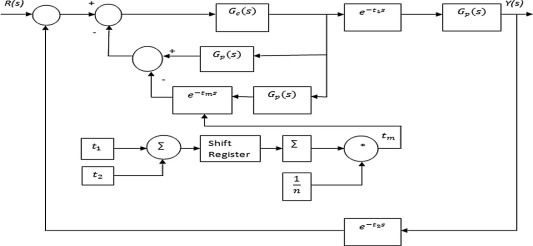Figure 4. The system with adaptive smith predictor.

## 6. Simulated results

In this paper, the classical and adaptive Smith predictors are applied to compensate the delay of the NCS using the IEEE 802.15.4 network. This section presents the performance evaluation of our scheme.

### 6.1. Markov model and network time delay

The IEEE 802.15.4 is modeled using Markov Chain model as shown in Section 4. Matlab Software is used to implement this model and the built-in function fsolve is used to solve its equations. The delay ${\textstyle \left({\overline {D}}\right)}$ is computed in terms of the number of slots. Using the default values of the IEEE 802.15.4 MAC layer constants, the data rate = 250 KbPS and the slot time = 0.96 ms , the time induced delay = ${\textstyle {\overline {D}}}$ ∗ slot time. Table 1 presents the computed delay when the network consists of one sensor, 2 sensors and 5 sensors.

Table 1. The network delay ${\textstyle \left({\overline {D}}\right)}$ and time induced delay.
Number of sensors ${\textstyle {\overline {D}}}$ (slot) Time induced delay (ms)
1 40 38.4
2 47 45.12
5 59 56.64

Table 1 shows that the computed delay increases by increasing the number of sensors that is due to the competition among the sensors in accessing the shared network. The number of transmission attempts ended by channel access failure, or by collision increases, so the network delay increases.

### 6.2. Delay compensation using Smith predictor

To evaluate the delay compensation using Smith predictor, a wireless DC-motor NCS using direct structure design approach is built to control the motor speed. The DC motor transfer function is given as follows:

 $G(s)={\frac {107}{S^{3}+11S^{2}+91S+108.3}}$
(17)

In our design, the plant consists of a sensor, an actuator and the DC motor. The controller is a discrete PID regulator, and its parameters are P = 1.2, I = 1.4, D = 0.1 and the sample time is 0.03 s . Each plant is connected to its controller using IEEE 802.15.4 wireless network. In the paper, the channel behavior is considered as uniform distribution. The wireless NCS is implemented using TrueTime tool based on Matlab/Simulink software.

Fig. 5 shows the wireless NCS system without delay compensation, and it consists of one sensor, one actuator and one PID controller. This system is simulated to evaluate the performance of the proposed delay compensation schemes using both classical and predictive Smith predictor. Fig. 6 shows a comparison between the reference signal and the output signal without and with delay compensation.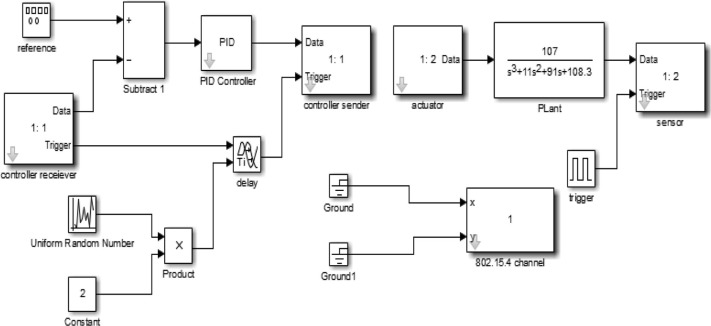Figure 5. Wireless NCS simulated model using one sensor without delay compensation.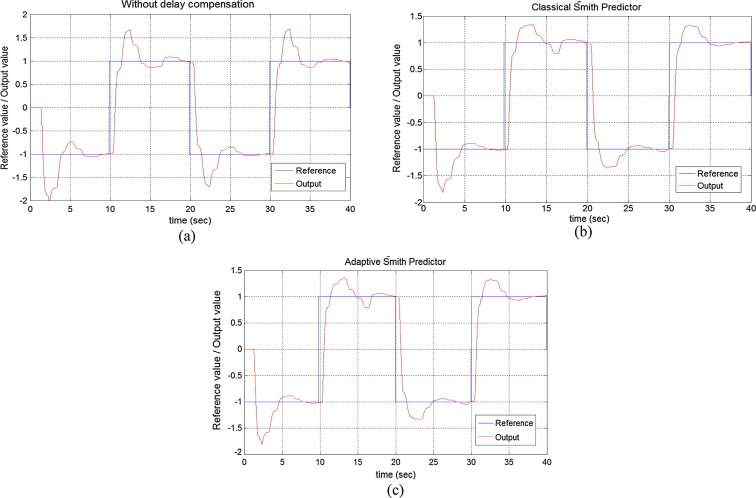Figure 6. The reference and the output signal in case of one sensor NCS: (a) Without delay compensation; (b) Delay compensation with classical Smith predictor; (c) Delay compensation with adaptive Smith predictor.

Fig. 6(a) shows that the network delay has a significant effect on the system, and the output signal is different from the reference signal. By applying the proposed delay compensation scheme, the difference between these two signals decreases and the output signal becomes closer to the reference one. It also shows that the output signals using classical smith predictor and adaptive smith predictor are very close, that is due to the use of constant delay based on the IEEE 802.15.4 Markov model.

In another experiment, three scenarios are implemented to study the effect of increasing the number of sensors on the system performance. The three scenarios are the wireless NCS with one sensor, two sensors, and five sensors. In each scenario, the system is simulated in three cases, without delay compensation, and with delay compensation using classical Smith predictor and adaptive smith predictor.

In this experiment, another performance metric is used to compare the reference and the output signal. This metric is the signal to error ratio (SER) given as

 ${\mbox{SER}}={\frac {\sum {\left(X(t)\right)}^{2}}{\sum {\left(X(t)-{\overset {\mbox{ˆ}}{X}}(t)\right)}^{2}}}$
(18)

where ${\textstyle X(t)}$ is the reference signal and ${\textstyle {\overset {\mbox{ˆ}}{X}}(t)}$ is the output speed of motor. Table 2 shows the SER of the three scenarios and the three cases.

Table 2. SER of the three scenarios.
Sensors Scenario
SER
Without delay compensation With delay compensation
Classical smith predictor Adaptive smith predictor
One sensor 3.2383 3.7387 3.7474
Two sensors Sensor 1 2.9877 3.3519 3.3575
Sensor 2 2.1955 2.7088 2.7432
Five sensors Sensor 1 2.6575 3.0280 3.0290
Sensor 2 1.8488 2.4114 2.4432
Sensor 3 2.8519 2.9962 2.9978
Sensor 4 1.7154 2.2646 2.2687
Sensor 5 2.3523 2.6995 2.7101

In the case of one-sensor system, Table 2 supports the same results where the SER increases by using the delay compensation scheme in all cases. It also shows that the value of SER in the case of classical smith predictor is close to the value of SER in the case of adaptive smith predictor in all scenarios.

Table 2 shows that as the number of sensors increases, the SER decreases. As the number of sensor increases, the competition among them to access the networks increases, and the network delay increases. The proposed delay compensation schemes result in improving the system performance for all cases. The adaptive Smith predictor scheme gives better performance compared to the classical scheme.

In another experiment, the proposed delay compensation schemes are compared to those schemes using Smith predictor proposed in , and . As proposed in , our classical Smith predictor scheme is modified to use the induced time as random value equal to ${\textstyle t_{m}=46{\mbox{ms}}}$. To compare with , our adaptive Smith Predictor is used without the shift register. Table 3 shows the comparison results in the scenario of one-sensor NCS.

Table 3. Comparison results in terms of SER.
Scheme Proposed Schemes Classical Smith predictor proposed in  Adaptive Smith predictor proposed in 
Classical Smith predictor Adaptive Smith predictor
SER 3.7387 3.7474 3.5208 3.6813

The results in Table 3 show that the schemes proposed in this paper improve the performance. They give better SER compared to the other schemes proposed in  and . The proposed classical Smith predictor uses accurate induced time value based on IEEE 802.15.4 Markov model instead of any random value. The proposed adaptive Smith predictor considers the channel behavior to estimate more accurately induced time delay.

## 7. Conclusions and future work

Wireless NCS relies on the IEEE 802.15.4 MAC protocol. This protocol results in time delay that may cause NCS instability. A delay compensation using classical and adaptive Smith predictors is proposed. In the Classical Smith predictor, the network delay is computed based on the IEEE 802.15.4 Markov model instead of using any random value. In the adaptive Smith predictor, the network delay is computed as the average value of the previous measured delay stored in discrete shift register. The system is evaluated by building a wireless DC-motor NCS using True-Time based Matlab/Simulink software. The results show that the proposed delay compensation schemes improve the performance in the case of one, two and five sensors. The proposed schemes give better performance compared to other schemes in the literature.

In the future work, the proposed Smith predictor schemes can be applied to different NCSs. Other delay compensation schemes can be applied and compared to the proposed ones.

### Document informationPublished on 12/04/17

Licence: Other

### Document Score0

Views 125
Recommendations 0